# mne.minimum_norm.make_inverse_resolution_matrix#

mne.minimum_norm.make_inverse_resolution_matrix(forward, inverse_operator, method='dSPM', lambda2=0.1111111111111111, verbose=None)[source]#

Compute resolution matrix for linear inverse operator.

Parameters:
forwardinstance of `Forward`

Forward Operator.

inverse_operatorinstance of `InverseOperator`

Inverse operator.

method‘MNE’ | ‘dSPM’ | ‘sLORETA’

Inverse method to use (MNE, dSPM, sLORETA).

lambda2`float`

The regularisation parameter.

verbose

Control verbosity of the logging output. If `None`, use the default verbosity level. See the logging documentation and `mne.verbose()` for details. Should only be passed as a keyword argument.

Returns:
resmat: `array`, shape (n_orient_inv * n_dipoles, n_orient_fwd * n_dipoles)

Resolution matrix (inverse operator times forward operator). The result of applying the inverse operator to the forward operator. If source orientations are not fixed, all source components will be computed (i.e. for n_orient_inv > 1 or n_orient_fwd > 1). The columns of the resolution matrix are the point-spread functions (PSFs) and the rows are the cross-talk functions (CTFs).

## Examples using `mne.minimum_norm.make_inverse_resolution_matrix`#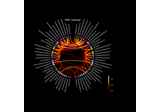Visualize source leakage among labels using a circular graph

Visualize source leakage among labels using a circular graph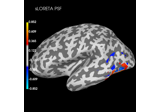Plot point-spread functions (PSFs) and cross-talk functions (CTFs)

Plot point-spread functions (PSFs) and cross-talk functions (CTFs)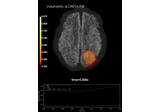Plot point-spread functions (PSFs) for a volume

Plot point-spread functions (PSFs) for a volume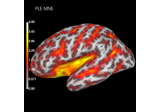Compute spatial resolution metrics in source space

Compute spatial resolution metrics in source space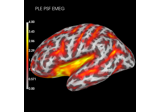Compute spatial resolution metrics to compare MEG with EEG+MEG

Compute spatial resolution metrics to compare MEG with EEG+MEG